# Variables

Use variables, which can be defined as a value or expression, instead of fixed values when defining the parameters of many geometry and the dimensions of sketching tools. When you change a value or expression, the model is updated accordingly.

## Make the Current Parameter a Variable

Make the current parameter a variable.

1. While editing the parameter, do one of the following:
• Enter a formula and press Enter. For example, if you enter L=3, the length becomes 3.0 cm and the variable L is created.
• Click f{x}, and then select Create Variable. The current value is assigned a variable name automatically.2. You can edit the variable in the Variable Manager or Design Table.
• In the Variable Manager, you can add, edit, and remove expressions.
• In the Design Table, you can change the value of numeric expressions (e.g., R=10 cm is a numeric expression, but R=2*3 cm and R=L+5 cm are not). You can also make these variables animation properties (i.e., objects whose values can change over time).

## Manage Variables

Use the Variable Manager to edit variables, create new variables, and import or export variables in .csv format.

1. On the ribbon, click the Analysis tab.
2. Click the Variables icon.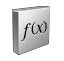The Variable Manager is displayed.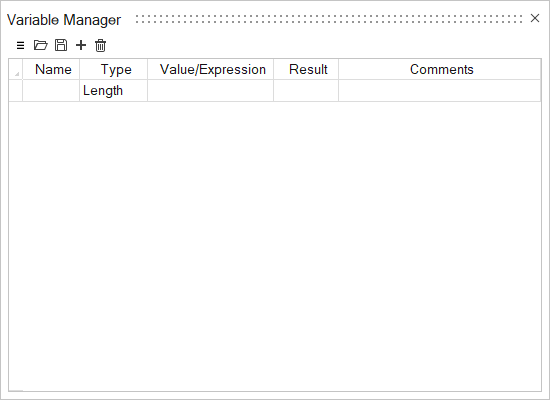3. You can do the following:
To Do this Notes
Import variables from a .csv file Click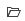. The required format is name,expression,type,comment where type is 0 = length, 4=angle, 1000=unitless, 2000=string.
Warning: When you import variables, all existing variables will be overwritten.
Export variables to a .csv file Click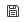.
Delete one or more variables
1. Select one or more variables.
2. Click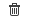1. Select an empty Name field or click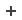, enter a name, and then press Enter.
Important: The Name field may not be left blank. A variable’s name may not be a math operator, numeric value, mathematical constant, reserved Python keyword, or a unit specifier. The name may include underscores (_), but no spaces or other special characters.
2. In the Type column, select a variable type:
• Length (default): This refers to not only length, but also height, width, and diameter.
• Angle
• String: This option is not currently supported in Inspire Studio.
• Unitless: Select this option for unitless parameters, like number of Copies in the Radial Symmetry tool.
3. In the Value/Expression column, enter a numerical value, or specify a dependent relationship between variables, e.g. OtherVariable*2. Basic math operators (+,-,/,*) and standard Python mathematical functions are accepted.

Inspire Studio automatically populates the Result field.

4. Optional: In the Comments column, enter any relevant notes.
If you specify units for a Length variable, e.g. 1.0 cm, then Inspire Studio will express the Result in the selected Display Units, e.g. 10 mm.

Multiplying a Length variable by another Length variable is unsupported because units squared (e.g. cm2) is unsupported.

Length variables and Angle variables may not be referenced in the same Expression.

You can use trigonometric functions in Expressions. If the input value is a variable, the variable must be unitless. For example, X = 1 (unitless) or H = 10*sin(X).

A variable’s Expression may not reference itself.

Circular dependencies, e.g. where the Expression for L1 refers to L2, the Expression for L2 refers to L3, and the Expression for L3 refers to L1, are not supported.

Non-length or non-angle (Mass, Force, etc.) units are currently unsupported.

Edit a variable Select the field you wish to edit and enter the new information.